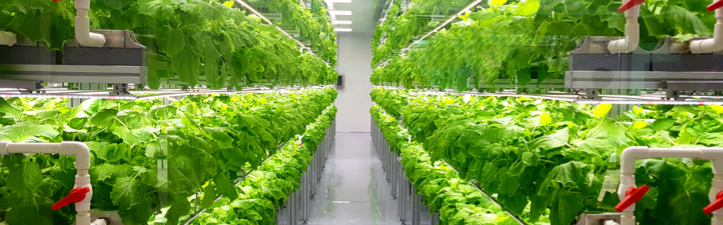# PyTorch: Tabular Classify Multi-Label

Categorizing Plant Species with Multi-Label Classification of Phenotypes.## 💾 Data

This dataset is comprised of:

• Label = the species of the plant.

• Features = phenotypes of the plant sample.

:

from aiqc import datum
df = datum.to_df('iris.tsv')

:

from aiqc.orm import Dataset
shared_dataset = Dataset.Tabular.from_df(df)
df.sample(3)

:

sepal_length sepal_width petal_length petal_width species
0 5.1 3.5 1.4 0.2 setosa
1 4.9 3.0 1.4 0.2 setosa
2 4.7 3.2 1.3 0.2 setosa

## 🚰 Pipeline

:

from aiqc.mlops import Pipeline, Input, Target, Stratifier
from sklearn.preprocessing import OrdinalEncoder, StandardScaler

:

pipeline = Pipeline(
Input(
dataset  = shared_dataset,
encoders = Input.Encoder(
StandardScaler(),
dtypes = ['float64']
)
),

Target(
dataset   = shared_dataset
, column  = 'species'
, encoder = Target.Encoder(OrdinalEncoder())
),

Stratifier(
size_test         = 0.09
, size_validation = 0.22
#, fold_count     = 5
)
)


└── Info - System overriding user input to set sklearn_preprocess.copy=False.
This saves memory when concatenating the output of many encoders.

Warning - The number of samples <117> in your training Split
is not evenly divisible by the fold_count <5> you specified.
This can result in misleading performance metrics for the last Fold.



## 🧪 Experiment

:

from aiqc.mlops import Experiment, Architecture, Trainer
import torch.nn as nn
from torch import optim
import torchmetrics as tm
from aiqc.utils.pytorch import fit


Note that num_classes is unique to PyTorch multi-classification.

:

def fn_build(
features_shape
, num_classes
, **hp
):
model = nn.Sequential(
# --- Input/Hidden Layer ---
nn.Linear(features_shape, hp['neurons'])
, nn.ReLU()
, nn.Dropout(p=0.3)

# --- Output Layer ---
, nn.Linear(hp['neurons'], num_classes)
, nn.Softmax(dim=1)
)
return model

:

def fn_train(
model
, loser
, optimizer

, train_features
, train_label
, eval_features
, eval_label

, **hp
):
model = fit(
model
, loser
, optimizer

, train_features
, train_label
, eval_features
, eval_label

, epochs     = hp['epochs']
, batch_size = hp['batch_size']
, metrics    = [tm.Accuracy(), tm.F1Score()]
)
return model

:

hyperparameters = dict(
batch_size   = 
, epochs     = [15,25]
, neurons    = [9,12]
, learn_rate = [0.01]
)

:

experiment = Experiment(
Architecture(
library           = "pytorch"
, analysis_type   = "classification_multi"
, fn_build        = fn_build
, fn_train        = fn_train
, hyperparameters = hyperparameters
),

Trainer(pipeline=pipeline, repeat_count=3)
)

[ ]:

experiment.run_jobs()


## 📊 Visualization & Interpretation

For more information on visualization of performance metrics, reference the Dashboard documentation.